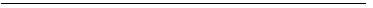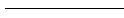Print View

[Section Index]

### The Standards of Weights and Measures Ordinance, 1982

#### ( Ordinance NO. XII OF 1982 )

Chapter I

STANDARD UNITS

Base units of System International
4. (1) The units of weights and measures to be used throughout Bangladesh shall be units known as system international (SI) units.

(2) For the purpose of sub section (1), the international system of units as recommended by the General Conference on Weights and Measures, and such additional units as may be recommended by the International Organisation of Legal Metrology shall be the units of the metric system or international system.

(3) The base units shall be as under-

(a) kilogram-

(i) the base unit of mass shall be the kilogram;

(ii) the “kilogram” is the unit of mass; it is equal to the mass of the international prototype of the kilogram;

(b) metre-

(i) the base unit of length shall be the metre;

1[(ii) the “metre” is the length of the path travelled by light in vacuum during a time interval of 1/299 792 458 of a second;]

(c) second-

(i) the base unit of time shall be a second;

(ii) a “second” is the duration of 9 192 631 770 periods of the radiation corresponding to the transition between the two hyperfine levels of the ground state of the caesium 133 atom;

(d) ampere-

(i) the base unit of electric current shall be the ampere;

(ii) the “ampere” is that constant current which, if maintained in two straight paralled conductors of infinite length, of negligible circular cross section, and placed one metre apart in vacuum, would produce between these conductors a force equal to 2 ├ù10 7┬¼ newton per metre of length;

(e) kelvin-

(i) the base unit of thermodynamic temperature shall be the kelvin;

(ii) the “kelvin” is the fraction 1/273.16 of the thermodynamic temperature of the triple point of water;

Note- (i) The unit kelvin and its symbol k will be used to express on interval or a difference of temperature:

(ii) In addition to the thermodynamic temperature (symbol t) expressed in kelvins use is also made of Celsius temperature (symbol t) defined by the equation t=T=To where To-273.15 k by definition. The Celsius temperature is in general expressed in “degrees Celsius” (symbol o C). The unit “degree Celsius” is thus equal to the unit “kelvin” and an interval or a difference of Celsius temperature may also be expressed in degrees Celsius:

(f) candela-

(i) the base unit of lumirous intensity shall be the candela;

(ii) the “candela” is the luminous intensity in the perpendicular direction, of a surface of 1/600 000 square metre of a black body at the temperature of freezing platinum under a pressure of 101 325 newtons per square metre;

(g) mole-

(i) the base unit of amount of substance shall be the mole;

(ii) the “mole” is the amount of substance of system which contains as many elementary entities as there are atoms in 0.012 kilogram of carbon 12.

Note.- When the mole is used, the elementary entities shall be specified and may be atoms, molecules, ions, electrons, other particle, or specified groups of such particles.• ###### 1
Sub-clause (ii) was substituted by section 3 of the Standards of Weights and Measures (Amendment) Act, 2001 (Act No. VI of 2001).